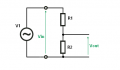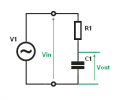# Dependence of Cut off frequency of RC circuit...

Status
Not open for further replies.

#### Himanshoo

Joined Apr 3, 2015
265
Hi guys ,
....my question is that why the cut off frequency of an RC circuit depends upon 'R'...according to formula
f = 1/ 2pi R C... Since as we all know that capacitor is frequency selective..not resistance ...so the cutoff frequency should be solely dependent on capacitance...as the resistance is indifferent to the frequency...also as per formula resistance is having its contribution ...why and how?

thanks

#### Jony130

Joined Feb 17, 2009
5,439
But F will also depend on C as well. Because we defined the cut off frequency as the frequency at which Xc = R. And this happens at frequencies equals Fc = 1/(2pi R C).

So for C = 1nF and R = 1kΩ we have :

Fc = 1/(2pi R C) ≈ 0.16/RC = 0.16/(1nF * 1kΩ) = 160kHz

But if we increase the capacitor ten times Fc will also drop ten times from 160kHz to 16kHz.

•Himanshoo

#### DickCappels

Joined Aug 21, 2008
9,625
The reason R is part of the expression is because the cutoff frequency is defined as the frequency at which the capacitive reactance is equal to the value of the resistance.

•Himanshoo

#### crutschow

Joined Mar 14, 2008
31,539
And when R = Xc the output of the RC network will be reduced by -3dB (0.707) hence it's also called the -3dB point.

•Himanshoo

#### MikeML

Joined Oct 2, 2009
5,444
•anhnha and Himanshoo

#### Himanshoo

Joined Apr 3, 2015
265
Because we defined the cut off frequency as the frequency at which Xc = R
Hi Jony..
very true said...but I am thinking like this...it makes no difference to a resistor whether an AC or a DC current is passing through it...as it behaves as the same to both of them..so its independent of the frequency.....so is it reasonable to say that cut of frequency depends on resistance as well.

#### Veracohr

Joined Jan 3, 2011
767
Yes, the cutoff frequency depends on the resistor as well. The cutoff frequency is defined as the frequency where the impedance of the resistor and the impedance of the capacitor are equal. If you change the resistor value, the frequency where they become equal changes as well, because the impedance of the capacitor is frequency dependent.

Just think of it is a voltage divider. The output of a voltage divider depends on the value of both impedances. That doesn't change if one of those is a frequency-dependent impedance.

•Himanshoo

#### Himanshoo

Joined Apr 3, 2015
265
And when R = Xc the output of the RC network will be reduced by -3dB (0.707) hence it's also called the -3dB point.
Most of the post says that Cut off frequency is something when Xc=R....ok...but i think so that they are talking of resonance as well....Iam not sure of RC circuit..but in an LC circuit resonance occurs when XL=Xc...so should cutoff frequency be thought of same as resonant frequency....? Hell they are different....

Talking about 3db rolloff ...its just due to the shunting effect of the capacitor ...which I earlier said is frequency selective...what role does 'R' has in it......?

#### crutschow

Joined Mar 14, 2008
31,539
The RC network is just a voltage divider with one element be a fixed impedance (the resistor) and one element with a varying impedance (the capacitor).
So the -3dB point will depend upon the relative impedance between the R and C.
Now the capacitor impedance is inversely proportional to frequency.
And it follows that changing either the value of R or C will change the frequency at which R = Xc, thus changing the -3dB point.

A simple RC circuit does not exhibit a resonance characteristic.
An LC circuit does.

#### Himanshoo

Joined Apr 3, 2015
265
The RC network is just a voltage divider with one element be a fixed impedance (the resistor) and one element with a varying impedance (the capacitor).
And it follows that changing either the value of R or C will change the frequency at which R = Xc, thus changing the -3dB point.
Ok i agree that -3db depends on relative impedance of R and C ...but what is the neccessity of making both of them equal (Xc=R) since RC circuit doesn't exhibit resonance..

Ok what I assume is that in order to roll off the gain(-3db) the output is shunted by the capacitor...now if the circuit is shunted by an RC circuit..then it requires both the impedance to be same(Xc=R) as not doing so will make the -3db point blurr (not sharp)...which could waste the bandwidth in question....
Now if I am thinking right... the question still remains the same as.. if sharpness of 3db point is our primary requirement then...It could alone be achieved by using a shunting capacitor ...then whats the use of involving 'R'...

#### kubeek

Joined Sep 20, 2005
5,776
Ok i agree that -3db depends on relative impedance of R and C ...but what is the neccessity of making both of them equal (Xc=R) since RC circuit doesn't exhibit resonance..
There is no necessity, it is just a convenient point.

Sharpness? And capacitor along with what, an ideal voltage source? You need to divide the voltage somehow, so you use two elements and one is typically a resistor and the other a capacitor.

•Himanshoo

#### MikeML

Joined Oct 2, 2009
5,444
•Himanshoo

#### Papabravo

Joined Feb 24, 2006
19,855
The ratio of resistance to capacitance that reduces the voltage across the capacitor to a prescribed value is unique. For any value of C there is exactly one vale of R that does the job. Conversely for any value of R there is exactly one value of C that does the job. No resonance, no smoke, and no mirrors. If both values are unspecified then you have a large set of combinations that will do the job.

#### Himanshoo

Joined Apr 3, 2015
265
So this circuit no longer work as a filter because Vin = Vout thanks to KVL.
Great Explanation.....Jony...

As you said that Vin= Vout when R is absent....so is it ok to consider any filter circuit as an 'attenuator'....??
So the function of 'R' is just to attenuate the Vin...? means to suppress unwanted frequencies....

#### Jony130

Joined Feb 17, 2009
5,439
Passive filter is is nothing more then a voltage divider, and divider is nothing more than a "attenuator".
If we build voltage divider using only two resistors we end-up with a circuit that will have a constant attenuation, and this attenuation does not change with frequency.
But if we build the voltage divider with capacitor and resistor we will have a filter. Because now attenuation will change together with frequency because R is unchanged but capacitor reactance Xc is changing with frequency.

•Himanshoo

#### Himanshoo

Joined Apr 3, 2015
265
But if we build the voltage divider with capacitor and resistor we will have a filter. Because now attenuation will change together with frequency because R is unchanged but capacitor reactance Xc is changing with frequency.
Actually it can be visualised as this way that..'R' acts as a valve which wont let Vout totally dependent on Vin...If Vout is totally dependent on Vin then....there wont be any attenuation as then Vin= Vout..and no -3db point.. since ..it can be thought ..in terms of filter that all of the Vin will appear at output..hence kind of passing all the frequencies...

But this inference of mine raise one more important question..that ..its fine that 3db point (which is also thought of as reduction in gain) depends upon the value of 'R'..and if all of the output is taken across C..then with frequency change in Vin will also changes Xc (load impedance)..which should further change the output voltage...and if this is the reason then ..it will not be wise to say that the rolloff point depends solely on the value of 'R'...
Since Mike simulation dont agree to this....
I am bit confused now...

#### Jony130

Joined Feb 17, 2009
5,439
OMG.

For this voltage dividerVout/Vin ( the gain) is constant regardless of the signal frequency, and this gain is equal to:

Vout = I*R2 = Vin/(R1 + R2) * R2 = Vin*R2/(R1 + R2)

Vout/Vin = R2/(R1 + R2)

For example if R1 = R2 = 1k , the gain is equal to 0.5V/V.

But now if we replace the R2 with capacitor we also have a voltage divider :But this time the voltage divide gain will change with frequency because Xc is changing with frequency.

$$Vout =I*Xc =\frac{Vin}{\sqr{ R_1^2 + X_{c1}^2}} * Xc$$

and Xc = 1/(2 * pi * F * C1)

So for Vin = 10V and F = 1kHz ; R1 = 1K and C1 = 160nF we have :

Xc = 1kΩ and Vout = 10V/√(1kΩ^2+1KΩ^2) * 1kΩ = 10V/√2MΩ * 1kΩ = 7.07mA * 1kΩ = 7.07V

But now if we change the signal frequency form 1kHz to 10kHz Vout will drop to, because now Xc is 100Ω not 1kΩ

Vout = 10V/√(1kΩ^2+100Ω^2) * 100Ω = 10V/√1.01MΩ * 100Ω = 9.95mA * 100Ω = 0.995V ≈ 1V.

#### Jony130

Joined Feb 17, 2009
5,439
As for fc and Xc = R, we have choose Fc at frequency where Xc = R because for F larger than Fc the gain start to drops -6dB per octave above Fc.
-6dB per octave means that every time the frequency is doubled the output voltage is halved.

Status
Not open for further replies.